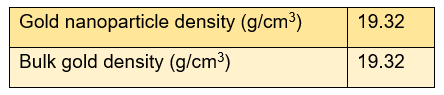#### How to Calculate the Volume, Mass, and Concentration of Gold Nanoparticle

Gold is a chemically stable metal with a molar mass of 196.96657 g/m and a density of 19.3 g/cm3. It is often used for decoration and money, but its properties change strangely when its size shrinks to the nanometer level. Gold nanoparticles have special physical and chemical properties different from bulk gold because of their small size effect, surface effect, quantum size effect, and macroscopic quantum tunneling effect.

Gold nanoparticles have the characteristics of high electron density, high dielectric properties, and high catalytic efficiency, and can combine with a variety of biological macromolecules without affecting their biological activity. Therefore, it has been widely used in disease diagnosis and treatment, as well as drug delivery and other aspects. The volume, mass, and concentration of gold nanoparticles are the basic characteristics of gold nanoparticles. We will describe how to calculate these parameters of spherical gold nanoparticles and gold nanorods.

• Calculation of the volume of gold nanoparticles

The gold nanoparticle’s volume is determined by measuring its dimensions. The particle’s dimensions are measured using a transmission electron microscope (TEM) to calculate volume, for instance.

The volume of spherical particles is V=4/3𝜋r3, where r represents the sphere’s radius.

The volume of rod-shaped particles, on the other hand, is V=𝜋r2l, where r represents the rod’s radius and l the length.

• Calculation of the mass of gold nanoparticles

Once the volume of nanoparticles is calculated, their mass can be determined by multiplying the volume by the density of the material (ρ), according to the formula m=Vρ. Typically, the density of nanomaterials is the same as the bulk density, however, for some materials like silica, the atomic structure differs from the bulk density. In these cases, a corrected density should be used. The nanoparticle density and bulk density of gold materials are presented in the following figure.• Calculation of the concentration of gold nanoparticles

To determine the concentration of gold nanoparticles, the first step is to calculate the total mass of gold in nanoparticle form within the solution. An estimate can be obtained by assuming that all reagents initially added are converted to gold nanoparticles, with no accounting for low reaction yield or processing loss. This assumption entails that all the added gold chloride is reduced to elemental gold. The direct measurement method for determining element concentration yields more precise results.

For instance, the concentration of gold in the purified gold nanoparticle solution is directly measured via ICP-MS. This measurement permits calculation of the nanoparticle number concentration by dividing the solution’s total mass by that of an individual nanoparticle — the formula being N=MC/m.

Where MC represents the mass concentration of elements and m is the mass of each individual nanoparticle. If the total mass concentration is measured in g/mL and the particle mass is presented in g/nps, the concentration can be calculated as nps/mL.

• Molar concentration of gold nanoparticles

In chemical and biological studies, molar concentration is typically used to express concentration and refers to the number of moles per liter of the substance. It is important to note that the molar concentration of particles differs from that of the elements that compose the nanoparticles. To calculate the molar concentration of particles, the formula M=N/NA is utilized, where N represents the number concentration of nanoparticles (in nps/L), and the denominator is the Avogadro constant (NA=6.02×1023).

The molar concentration of gold nanoparticles is generally in the range of nM to pM. For example, our DiagNano™ Gold Nanoparticle, 20 nm has a total elemental gold concentration of 0.0483 mg/mL, a corresponding particle number concentration of 6.82×1011 nps/mL, and a particle molarity of 1.14 nM.

CD Bioparticles provides online conversion tools to help customers convert parameters such as the volume, mass, and concentration of gold nanoparticles.

• 50+ DA New Bookmarking Sites
•www.smallbookmarking.com
•www.dbookmarking.com
•www.aibookmarking.com
•www.smallbookmarking.com
•www.newsbookmarking.com
•www.bbookmarking.com
•www.webbookmarks.hiauthors.com
• webbookmarks.hiauthors.com